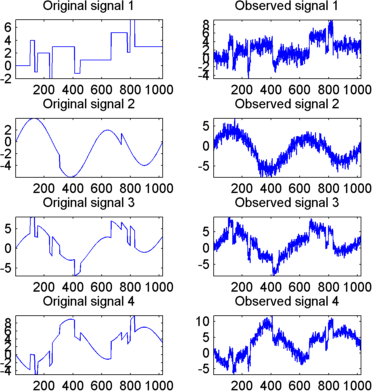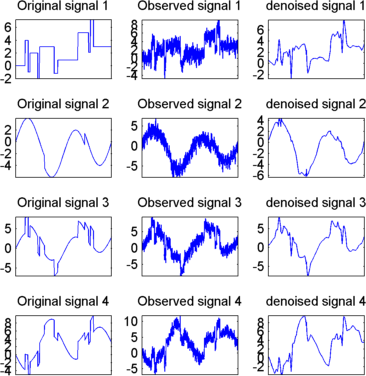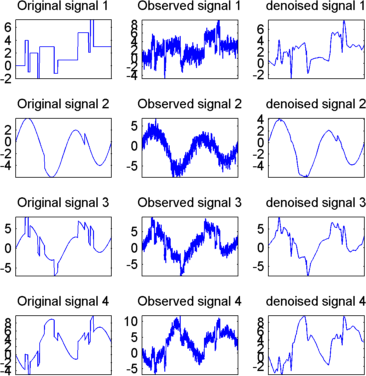## Multivariate Wavelet Denoising

This section demonstrates the features of multivariate denoising provided in the Wavelet Toolbox™ software. The toolbox includes the `wmulden` function and a Wavelet Analyzer app . This section also describes the command-line and app methods and includes information about transferring signal and parameter information between the disk and the app.

This multivariate wavelet denoising problem deals with models of the form X(t) = F(t) + e(t), where the observation X is p-dimensional, F is the deterministic signal to be recovered, and e is a spatially correlated noise signal. This kind of model is well suited for situations for which such additive, spatially correlated noise is realistic.

### Multivariate Wavelet Denoising — Command Line

This example uses noisy test signals. In this section, you will

• Display the original and observed signals.

• Remove noise by a simple multivariate thresholding after a change of basis.

• Display the original and denoised signals.

• Improve the obtained result by retaining less principal components.

• Display the number of retained principal components.

• Display the estimated noise covariance matrix.

1. Load a multivariate signal by typing the following at the MATLAB® prompt:

```load ex4mwden whos ```
NameSizeBytesClass
`covar``4x4``128``double array`
`x``1024x4``32768``double array`
`x_orig``1024x4``32768``double array`

Usually, only the matrix of data `x` is available. Here, we also have the true noise covariance matrix (`covar`) and the original signals (`x_orig`). These signals are noisy versions of simple combinations of the two original signals. The first one is “Blocks” which is irregular, and the second is “HeavySine,” which is regular except around time 750. The other two signals are the sum and the difference of the two original signals. Multivariate Gaussian white noise exhibiting strong spatial correlation is added to the resulting four signals, which leads to the observed data stored in `x`.

2. Display the original and observed signals by typing

```kp = 0; for i = 1:4 subplot(4,2,kp+1), plot(x_orig(:,i)); axis tight; title(['Original signal ',num2str(i)]) subplot(4,2,kp+2), plot(x(:,i)); axis tight; title(['Observed signal ',num2str(i)]) kp = kp + 2; end ```The true noise covariance matrix is given by

```covar covar = 1.0000 0.8000 0.6000 0.7000 0.8000 1.0000 0.5000 0.6000 0.6000 0.5000 1.0000 0.7000 0.7000 0.6000 0.7000 1.0000 ```
3. Remove noise by simple multivariate thresholding.

The denoising strategy combines univariate wavelet denoising in the basis where the estimated noise covariance matrix is diagonal with noncentered Principal Component Analysis (PCA) on approximations in the wavelet domain or with final PCA.

First, perform univariate denoising by typing the following to set the denoising parameters:

```level = 5; wname = 'sym4'; tptr = 'sqtwolog'; sorh = 's'; ```

Then, set the PCA parameters by retaining all the principal components:

```npc_app = 4; npc_fin = 4; ```

Finally, perform multivariate denoising by typing

```x_den = wmulden(x, level, wname, npc_app, npc_fin, tptr, sorh); ```
4. Display the original and denoised signals by typing

```kp = 0; for i = 1:4 subplot(4,3,kp+1), plot(x_orig(:,i)); set(gca,'xtick',[]); axis tight; title(['Original signal ',num2str(i)]) subplot(4,3,kp+2), plot(x(:,i)); set(gca,'xtick',[]); axis tight; title(['Observed signal ',num2str(i)]) subplot(4,3,kp+3), plot(x_den(:,i)); set(gca,'xtick',[]); axis tight; title(['denoised signal ',num2str(i)]) kp = kp + 3; end ```5. Improve the first result by retaining fewer principal components.

The results are satisfactory. Focusing on the two first signals, note that they are correctly recovered, but the result can be improved by taking advantage of the relationships between the signals, leading to an additional denoising effect.

To automatically select the numbers of retained principal components by Kaiser's rule (which keeps the components associated with eigenvalues exceeding the mean of all eigenvalues), type

```npc_app = 'kais'; npc_fin = 'kais'; ```

Perform multivariate denoising again by typing

```[x_den, npc, nestco] = wmulden(x, level, wname, npc_app, ... npc_fin, tptr, sorh); ```
6. Display the number of retained principal components.

The second output argument gives the numbers of retained principal components for PCA for approximations and for final PCA.

```npc npc = 2 2 ```

As expected, since the signals are combinations of two initial ones, Kaiser's rule automatically detects that only two principal components are of interest.

7. Display the estimated noise covariance matrix.

The third output argument contains the estimated noise covariance matrix:

```nestco nestco = 1.0784 0.8333 0.6878 0.8141 0.8333 1.0025 0.5275 0.6814 0.6878 0.5275 1.0501 0.7734 0.8141 0.6814 0.7734 1.0967 ```

As you can see by comparing with the true matrix covar given previously, the estimation is satisfactory.

8. Display the original and final denoised signals by typing

```kp = 0; for i = 1:4 subplot(4,3,kp+1), plot(x_orig(:,i)); set(gca,'xtick',[]); axis tight; title(['Original signal ',num2str(i)]); set(gca,'xtick',[]); axis tight; subplot(4,3,kp+2), plot(x(:,i)); set(gca,'xtick',[]); axis tight; title(['Observed signal ',num2str(i)]) subplot(4,3,kp+3), plot(x_den(:,i)); set(gca,'xtick',[]); axis tight; title(['denoised signal ',num2str(i)]) kp = kp + 3; end ```The results are better than those previously obtained. The first signal, which is irregular, is still correctly recovered, while the second signal, which is more regular, is denoised better after this second stage of PCA.

### Multivariate Wavelet Denoising Using the Wavelet Analyzer App

This section explores a denoising strategy for multivariate signals using the Wavelet Analyzer app.

1. Start the Multivariate Denoising Tool by first opening the Wavelet Analyzer app. Type `waveletAnalyzer` at the command line.2. Click Multivariate Denoising to open the Multivariate Denoising portion of the app.

At the MATLAB command prompt, type

`load ex4mwden`
In the Multivariate Denoising tool, select File > Import from Workspace. When the Import from Workspace dialog box appears, select the `x` variable. Click OK to import the noisy multivariate signal. The signal is a matrix containing four columns, where each column is a signal to be denoised.These signals are noisy versions from simple combinations of the two original signals. The first one is “Blocks” which is irregular and the second is “HeavySine” which is regular except around time 750. The other two signals are the sum and the difference between the original signals. Multivariate Gaussian white noise exhibiting strong spatial correlation is added to the resulting four signals.

The following example illustrates the two different aspects of the proposed denoising method. First, perform a convenient change of basis to cope with spatial correlation and denoise in the new basis. Then, use PCA to take advantage of the relationships between the signals, leading to an additional denoising effect.

4. Perform a wavelet decomposition and diagonalize the noise covariance matrix.

Use the displayed default values for the Wavelet, the DWT Extension Mode, and the decomposition Level, and then click Decompose and Diagonalize. The tool displays the wavelet approximation and detail coefficients of the decomposition of each signal in the original basis.Select Noise Adapted Basis to display the signals and their coefficients in the noise-adapted basis.Eigenvectors define the change of basis, and eigenvalues are the variances of uncorrelated noises in the new basis.

The multivariate denoising method proposed below is interesting if the noise covariance matrix is far from diagonal exhibiting spatial correlation, which, in this example, is the case.

5. denoise the multivariate signal.

A number of options are available for fine-tuning the denoising algorithm. However, we will use the defaults: fixed form soft thresholding, scaled white noise model, and the proposed numbers of retained principal components. In this case, the default values for PCA lead to retaining all the components.

Select Original Basis to return to the original basis and then click Denoise.The results are satisfactory. Both of the two first signals are correctly recovered, but they can be improved by getting more information about the principal components. Click More on Principal Components.A new figure displays information to select the numbers of components to keep for the PCA of approximations and for the final PCA after getting back to the original basis. You can see the percentages of variability explained by each principal component and the corresponding cumulative plot. Here, it is clear that only two principal components are of interest.

Close the More on Principal Components window. Select `2` as the Nb. of PC for APP. Select `2` as the Nb. of PC for final PCA, and then click denoise.The results are better than those previously obtained. The first signal, which is irregular, is still correctly recovered. The second signal, which is more regular, is denoised better after this second stage of PCA. You can get more information by clicking Residuals.

### Importing and Exporting from the Wavelet Analyzer App

The tool lets you save denoised signals to disk by creating a MAT-file in the current folder with a name of your choice.

To save the signal denoised in the previous section,

1. Select File > Save denoised Signals.

2. Select Save denoised Signals and Parameters. A dialog box appears that lets you specify a folder and filename for storing the signal.

3. Type the name `s_ex4mwden` and click OK to save the data.

```load s_ex4mwdent whos ```
NameSizeBytesClass
`DEN_Params``1x1``430``struct array`
`PCA_Params``1x1``1536``struct array`
`x``1024x4``32768``struct array`

The denoised signals are in matrix `x`. The parameters (`PCA_Params` and `DEN_Params`) of the two-stage denoising process are also available.

• PCA_Params are the change of basis and PCA parameters:

```PCA_Params PCA_Params = NEST: {[4x4 double] [4x1 double] [4x4 double]} APP: {[4x4 double] [4x1 double] } FIN: {[4x4 double] [4x1 double] } ```

`PCA_Params.NEST{1}` contains the change of basis matrix. `PCA_Params.NEST{2}` contains the eigenvalues, and `PCA_Params.NEST{3}` is the estimated noise covariance matrix.

`PCA_Params.APP{1}` contains the change of basis matrix, `PCA_Params.APP{2}` contains the eigenvalues, and `PCA_Params.APP{3}` is the number of retained principal components for approximations.

The same structure is used for `PCA_Params.FIN` for the final PCA.

• `DEN_Params` are the denoising parameters in the diagonal basis:

```DEN_Params DEN_Params = thrVAL: [4.8445 2.0024 1.1536 1.3957 0] thrMETH: 'sqtwolog' thrTYPE: 's' ```

The thresholds are encoded in `thrVAL`. For `j` from `1` to `5`, `thrVAL(j) `contains the value used to threshold the detail coefficients at level `j`. The thresholding method is given by `thrMETH` and the thresholding mode is given by `thrTYPE`.

## SupportGet trial now